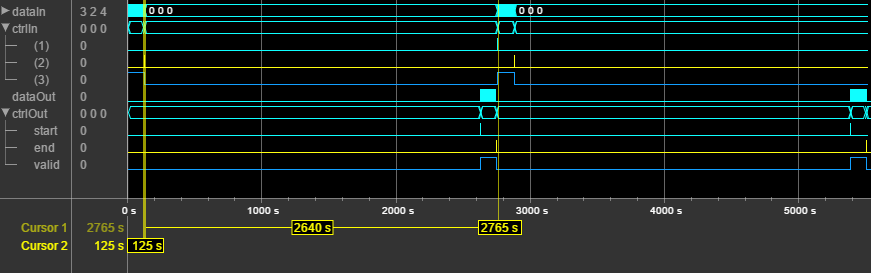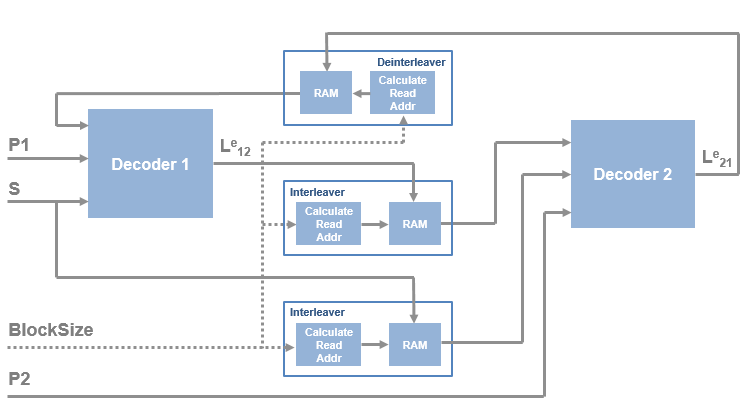# LTE Turbo Decoder

Decode turbo-encoded samples

•Libraries:
Wireless HDL Toolbox / Error Detection and Correction

## Description

The LTE Turbo Decoder block implements the turbo decoder required by LTE standard TS 36.212  and provides an interface and architecture optimized for HDL code generation and hardware deployment. The block iterates over two MAX decoders. You can specify the number of iterations. The coding rate is 1/3. The block accepts encoded bits as a 3-by-1 vector of soft-coded values, `[S P1 P2]`. In this vector, `S` is the systematic bit, and `P1` and `P2` are the parity bits from the two encoders.

This block uses a streaming sample interface with a bus for related control signals. This interface enables the block to operate independently of frame size, and to connect easily with other Wireless HDL Toolbox™ blocks. The block accepts and returns a value representing a single sample, and a bus containing three control signals. These signals indicate the validity of each sample and the boundaries of the frame. To convert a matrix into a sample stream and these control signals, use the Frame To Samples block or the `whdlFramesToSamples` function. For a full description of the interface, see Streaming Sample Interface.

The block can accept the next frame only after it has completed decoding the previous frame. You must leave Iterations*2*HalfIterationLatency+BlockSize+4 idle cycles between input frames. The half-iteration latency is described in the Algorithms section. Alternatively, you can use the output signal ctrl.`end` to determine when the block is ready for new input.

This waveform shows an input frame of 120 samples (+ 4 tail bits), and 2632 idle cycles between frames. Each input sample is a vector of three fixed-point soft-decision values. The input and output ctrl buses are expanded to show the control signals. `start` and `end` show the frame boundaries, and `valid` qualifies the data samples.## Ports

### Input

expand all

Input sample, specified as a three-element integer vector. The values represent soft-coded probabilities. If the value is negative, the bit is more likely to be 0. If the value is positive, the bit is more likely to be 1. The first element is the sequential bit, and the other two elements are parity bits. The block expects input frames of BlockSize + 12 samples. This frame size includes the tail bits, in the order specified by LTE standard TS 36.212 .

For a hardware implementation, use a fixed-point type with two or three integer bits and one to four fractional bits. Internal data types are derived from this data type, and lower precision types can result in loss of decoding precision. If the input data type has zero fractional bits or less than two integer bits, the block returns a warning. `double` and `single` are supported for simulation but not for HDL code generation.

Data Types: `fixdt(1,WL,FL)` | `single` | `double`

Control signals accompanying the sample stream, specified as a `samplecontrol` bus. The bus includes the `start`, `end`, and `valid` control signals, which indicate the boundaries of the frame and the validity of the samples.

• `start` — Indicates the start of the input frame

• `end` — Indicates the end of the input frame

• `valid` — Indicates that the data on the input data port is valid

For more details, see Sample Control Bus.

Data Types: `bus`

Turbo code block size, specified as an integer. This value must be one of the 188 values specified in the LTE standard, from 40 to 6144 in these intervals: ```[40:8:512 528:16:1024 1056:32:2048 2112:64:6144]```.

#### Dependencies

This port appears when you set Block size source to `Input port`.

Data Types: `single` | `double` | `uint16` | `fixdt(0,13,0)`

### Output

expand all

Output sample, returned as a binary scalar. `double` and `single` are supported for simulation but not for HDL code generation.

Data Types: `single` | `double` | `Boolean` | `ufix1`

Control signals accompanying the sample stream, returned as a `samplecontrol` bus. The bus includes the `start`, `end`, and `valid` control signals, which indicate the boundaries of the frame and the validity of the samples.

• `start` — Indicates the start of the output frame

• `end` — Indicates the end of the output frame

• `valid` — Indicates that the data on the output data port is valid

For more details, see Sample Control Bus.

Data Types: `bus`

## Parameters

expand all

Select whether you specify the block size with an input port or enter a fixed value as a parameter. If you select `Property`, the Block size parameter appears. If you select ```Input port```, the blockSize port appears.

Turbo code block size, specified as an integer. This value must be one of the 188 values specified in the LTE standard, from 40 through 6144 in these intervals: ```[40:8:512 528:16:1024 1056:32:2048 2112:64:6144]```. This value is registered for each frame, when ctrl.`start` = 1 (`true`).

#### Dependencies

This parameter appears when you set Block size source to `Property`.

How many MAX decoding iterations to perform, specified as a positive integer. The decoder implementation uses a single MAX decoder, with the output data of each half-iteration routed back to the input. A higher iteration count increases accuracy and adds latency. After about 15 iterations, the algorithm does not provide further accuracy.

## Algorithms

expand all

The block implements an iterative decode algorithm using a single decoder and single interleaver.

This diagram shows the conceptual algorithm for one iteration. Although the diagram shows two decoders and three interleavers, the block actually implements the algorithm using only one decoder and one interleaver. The decoder performs one half-iteration and interleaves the results. Then the output is routed back to the input for the next half-iteration. The interleaver computes the interleave indexes from the block size. For details of the interleaver implementation, see LTE Turbo Encoder.The odd half-iterations compute the likelihood ratio from uninterleaved bits (P1, S, and deinterleaved results of P2 decoding). The even half-iterations compute the likelihood ratio from the interleaved bits (P2 and interleaved results of P1 and S decoding).

The decoder block uses the BCJR algorithm to find the likelihood ratio of a particular bit .

The probabilities can also be represented in terms of current and future states:

The α probability represents the previous state, β represents the current state probability, and ɣ represents the next state. The algorithm calculates ɣ from the input values. The α and β probabilities are calculated using forward and backward recursion over the possible states of the trellis, and also depend on ɣ. All calculations are done in the `log` domain.

The initial conditions for α and β are:

`$\begin{array}{l}{\alpha }_{0}\left(s\right)=\left\{\begin{array}{c}1,s=0\\ 0,s\ne 0\end{array}\\ {\beta }_{0}\left(s\right)=\left\{\begin{array}{c}1,s=0\\ 0,s\ne 0\end{array}\end{array}$`

This diagram shows the half-iteration decoder and interleaver architecture. The initial likelihood is set to zero.In a normal BCJR architecture, the algorithm cannot compute β until the entire frame is in memory. It must perform a full-frame forward trace and then a full-frame backward trace, which means the latency of one half-iteration is two frame lengths. The required memory is `BlockSize*NumStates*DataWidth`. In this case, NumStates is eight, from LTE standard TS 36.212 .

However, this decoder implementation uses a sliding window to reduce the required memory and the latency of the algorithm . The window size is 32 samples, which is five times the trellis constraint length of 7. The latency of one half-iteration is:

`$\left(ceil\left(\frac{BlockSize}{WinSize}\right)+2\right)×WinSize+PipeDelay$`

The required memory with sliding window is 2*32*NumStates*DataWidth. This figure shows how the β calculation traces and decodes one window at a time, alternating input between the A and B calculation blocks.3GPP TS 36.212. "Multiplexing and channel coding." 3rd Generation Partnership Project; Technical Specification Group Radio Access Network; Evolved Universal Terrestrial Radio Access (E-UTRA). URL: https://www.3gpp.org.

 Bahl, L. R., J. Cocke, F. Jelinek, and J. Raviv. "Optimal Decoding of Linear Codes for Minimizing Symbol Error Rate." IEEE Transactions on Information Theory. Vol 1T-20, March 1974, pp. 284–287.

 Viterbi, Andrew J. "An Intuitive Justification and a Simplified Implementation of the MAP Decoder for Convolutional Codes." IEEE Journal on Selected Areas in Communications. Vol. 16, No. 2, February 1998.